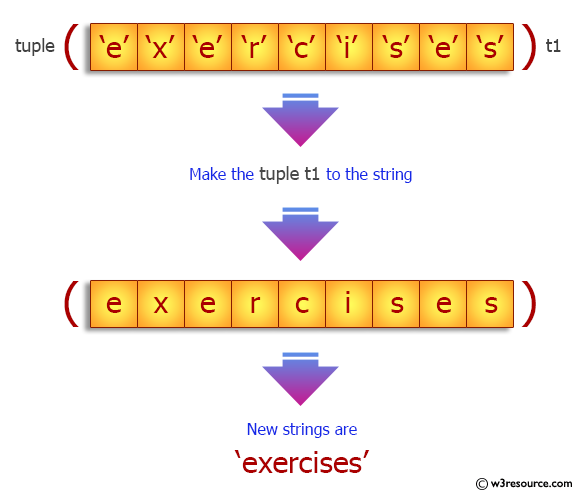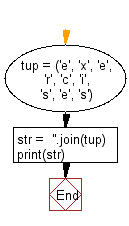﻿ Python: Tuple to a string - w3resource# Python Exercise: Tuple to a string

## Python tuple: Exercise-6 with Solution

Write a Python program to convert a tuple to a string.

Sample Solution:-

Python Code:

``````tup = ('e', 'x', 'e', 'r', 'c', 'i', 's', 'e', 's')
str =  ''.join(tup)
print(str)
```
```

Sample Output:

```exercises
```

Pictorial Presentation:Flowchart:## Visualize Python code execution:

The following tool visualize what the computer is doing step-by-step as it executes the said program:

Python Code Editor:

Have another way to solve this solution? Contribute your code (and comments) through Disqus.

What is the difficulty level of this exercise?

Test your Programming skills with w3resource's quiz.

﻿

## Python: Tips of the Day

Decapitalizes the first letter of a string:

Example:

```def tips_decapitalize(s, upper_rest=False):
return s[:1].lower() + (s[1:].upper() if upper_rest else s[1:])
print(tips_decapitalize('PythonTips'))
print(tips_decapitalize('PythonTips', True))
```

Output:

```pythonTips
pYTHONTIPS
```